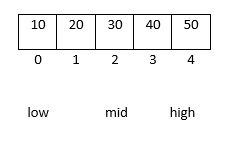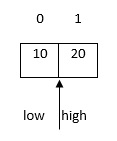# How to find minimum element in an array using binary search in C language?

C programming language provides two types of searching techniques. They are as follows −

• Linear search
• Binary search

## Binary Search

• This method can be applied only to sorted list.
• The given list is divided into two equal parts.
• The given key is compared with the middle element of the list.

Here, three situations may occur, which are as follows −

• If the middle element matches the key, then the search will end successfully here

• If the middle element is greater than the key, then the search will proceed in the left partition.

• If the middle element is lower than the key, then the search will proceed in the right partition.

Input (i/p) − Unsorted list of elements, key.

Output (o/p)

• Success – If key is found
• Unsuccessful – Otherwisekey = 20
mid = (low +high) /2## Program1

Following is the C program to find the minimum element in an array by using binary search −

#include<stdio.h>
int main(){
int a, n, i, key, flag = 0, low, mid, high;
printf("enter the no: of elements:");
scanf ("%d",&n);
printf("enter the elements:");
for(i=0; i<n; i++)
scanf( "%d", &a[i]);
printf("enter a key element:");
scanf ("%d", &key);
low = 0;
high = n-1;
while (low<= high ){
mid = (low + high) /2;
if (a[mid] == key){
flag = 1;
break;
}
else{
if (a[mid] > key)
high = mid-1;
else
low = mid+1;
}
}
if (flag == 1)
printf ("search is successful");
else
printf("search is unsuccessful");
return 0;
}

## Output

When the above program is executed, it produces the following result −

Run 1:
enter the no: of elements:5
enter the elements:
12
34
11
56
67
enter a key element:45
search is unsuccessful
Run 2:
enter the no: of elements:3
enter the elements:
12
34
56
enter a key element:34
search is successful

## Program2

Given below is another C program to find the minimum element in an array by using binary search −

#include<stdio.h>
void Bmin(int *a, int i, int n){
int j, temp;
temp = a[i];
j = 2 * i;
while (j <= n){
if (j < n && a[j+1] > a[j])
j = j + 1;
if (temp < a[j])
break;
else if (temp >= a[j]){
a[j / 2] = a[j];
j = 2 * j;
}
}
a[j/2] = temp;
return;
}
int binarysearchmin(int *a,int n){
int i;
for(i = n/2; i >= 1; i--){
Bmin(a,i,n);
}
return a;
}
int main(){
int n, i, x, min;
int a;
printf("Enter no of elements in an array");
scanf("%d", &n);
printf("Enter %d elements: ", n);
for (i = 1; i <= n; i++){
scanf("%d", &a[i]);
}
min = binarysearchmin(a, n);
printf("\minimum element in an array is : %d", min);
return 0;
}

## Output

When the above program is executed, it produces the following result −

Enter no of elements in an array
5
Enter 5 elements:
12
23
34
45
56
minimum element in an array is: 12